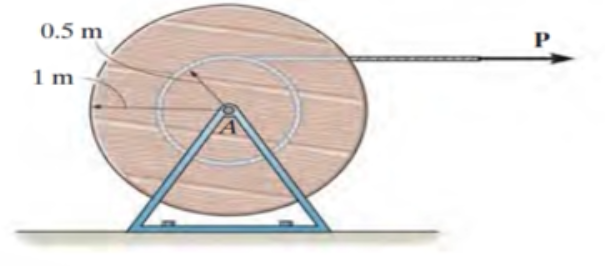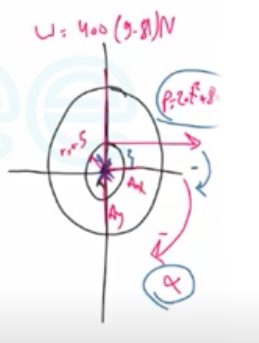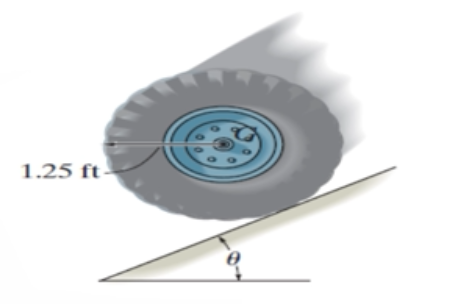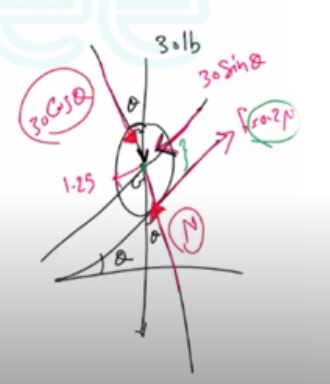Need Help?

Subscribe to Dynamic

###### \${selected_topic_name}
• Notes

$\begin{array}{l}{17-71 . \text { The reel of cable has a mass of } 400 \mathrm{kg} \text { and a radius }} \\ {\text { of gyration of } k_{A}=0.75 \mathrm{m} . \text { Determine its angular velocity }} \\ {\text { when } t=2 \mathrm{s} \text { , starting from rest, if the force } \mathbf{P}=\left(20 t^{2}+80\right) \mathrm{N} \text { , }} \\ {\text { when } t \text { is in seconds. Neglect the mass of the unwound cable, }} \\ {\text { and assume it is always at a radius of } 0.5 \mathrm{m} \text { . }}\end{array}$$m=400 kg$

$k_{A}=0.75 \mathrm{m}$

$\omega=??$

$@ t=2 s$

$P=\left(2 0t^{2}+80\right) N$

$r=0.5 m$

$I_{A}=MK_{A}^{2}=4 0 0(0.75)^{2}=225 \mathrm{kg} \cdot \mathrm{m}^{2}$$\Sigma { M_ A}=I_{A} \alpha$

$-\left(20 t^{2}+80\right)(0.5)=225(-\alpha)$

$∴ \alpha=\frac{2}{45}\left(t^{2}+4\right) rad / s^{2}$

$d \omega=\alpha d t \Rightarrow \omega=0 \ @ t=0$

$\int_{0}^{\omega} d \omega=\int_{0}^{2} \frac{2}{45}\left(t^{2}+4\right) d t$

$\omega=0.4741 \mathrm{rad} / \mathrm{s}=0.474 \mathrm{rad} / \mathrm{s}$

$\begin{array}{l}{\text { 17-95. The tire has a weight of } 301 \mathrm{b} \text { and a radius of }} \\ {\text { gyration of } k_{G}=0.6 \mathrm{ft} \text { . If the coefficients of static and }} \\ {\text { kinetic friction between the tire and the plane are } \mu_{s}=0.2} \\ {\text { and } \mu_{k}=0.15 \text { , determine the maximum angle } \theta \text { of the }} \\ {\text { inclined plane so that the tire rolls without slipping. }}\end{array}$$w=30lb$

$k_{G}=0.6 \mathrm{ft}$

$r=1.25 \mathrm{ft}$

$\mu_{s}=0.2 \ , \mu_k=0.15$$\Sigma F_{x}=m\left(a_{G}\right)_{x}$

$30 \sin \theta-0.2 N=\left(\frac{30}{32.2}\right)(1.25 \alpha) \rightarrow (1)$

$+\uparrow \Sigma F_y=m(a_{G})_{y}$

$N- 30 \cos \theta=0$

$N=30 \mathrm{cos} \theta$

$\Sigma M_{G}=I_{G} \alpha \Rightarrow 0.2 N(1.25)=\left[\left(\frac{30}{32.2}\right)(0.6^2)\right]\alpha$
$\alpha=0.745 \mathrm{N}$

$30 \sin \theta-6 \cos \theta=26.042 \cos \theta \Rightarrow 30 \sin \theta=32.042 \cos \theta$

$\tan \theta=1.068 \Rightarrow \theta-46.9^{\circ}$